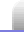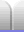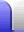# Key Words in Maths Algebra Exams

Some questions in algebra exams use key words which tell students what is required.

### Simplify

• Combine like terms (terms which have the same variables).
• Cancel common factors in fractions. This is easier to do when writing fractions with horizontal lines. (Remember that dividing by a number is the same as multiplying by its reciprocal.)

2xy - 3x + 2xy = 4xy -3x

4ab/2d ÷ 2a/d
= 4ab/2d × d/2a
= b

### Expand

• Remove brackets.
• You should then simplify if required.

(2x + 3y)(5x - 4y)
= 10x2 - 8xy + 15xy - 12y2
= 10 x2 + 7xy - 12y2

### Factorise

• Convert to a product of factors (write it using brackets).
 2x2 - 6x = 2x(x - 3) 4x2 - 16 4(x2 - 4) 4(x + 2)(x - 2)

### Evaluate

• Find the value of an expression (sometimes by substituting values in for variables in the expression).
• Not the same as solve.

Evaluate |z - x| where x = 6, z = -2
|-2 - 6| = |-8| = 8

√(26) = √(23 x 23) = 23 = 8

### Solve

• Find possible values for the variable(s).
• Simultaneous equations will have two or more variables to be solved.
• May involve expanding (and simplifying) or factorising.
• Not the same as evaluate.
 x2 + 12 = 7x ⇒ x2 - 7x + 12 = 0 (x - 3)(x - 4) = 0 ⇒ x - 3 = 0 or x - 4 = 0 ⇒ x = 3 or x = 4 (x - 4)/2 + (2x - 5)/3 = 1 ⇒ 3(x - 4) + 2(2x - 5) = 6 ⇒ 3x - 12 + 4x - 10 = 6 ⇒ 7x - 22 = 6 ⇒ 7x = 28 ⇒ x = 4

### Rearrange

• Make a particular variable the subject of an equation.
• The variable ends up on one side of the equation and everything else is on the other side of the equation.
• The variable is said to be expressed in terms of everything else.

Rearrange to make t the subject in s = ½at2
t2 = 2s/a
t = √(2s/a)

Being good at rearranging equations is very useful in physics.

### Sketch

• Draw a graph as neatly as possible.
• Perfect accuracy is not expected but key points should be accurate.
• There will likely be some known points or key points, such as where the graph intercepts the x and y axes, or the minimum/maximum point of a parabola.
• Often used with label.

### Label

• Write the equation of a graphed line in a convenient location next to the line.
• Often used with sketch.Home Astronomy Chemistry Electronics & Computers Mathematics Physics Field Trips Turn on javascript for email link